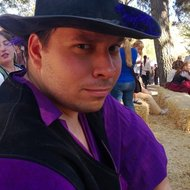# A rogue, and a scallywag

Probability Level 1

Bradan is thinking of a positive integer. The integer is either 3 digits with the last two digits equal to 21, or the integer is 2 digits with the first digit equal to 2. How many different integers could Bradan be thinking of?Details and assumptions

The number $12=012$ is a 2-digit number, not a 3-digit number.

×